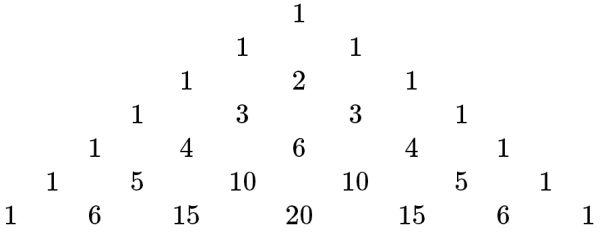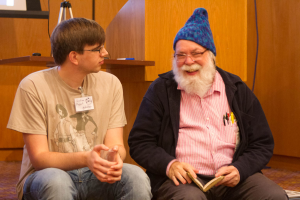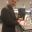# Open Season – Singmaster’s Conjecture

Science and maths are all about finding things out. Mathematics in particular is about making statements, and then determining their truth (or falsity). Finding a proof, or disproof, of a mathematical theory can be as simple as finding a counterexample, or it can take hundreds of authors tens of thousands of pages.

In this short series of articles, I’m going to write about some mathematical questions we don’t know the answer to – which haven’t yet been proven or disproven. Hopefully you will find it interesting, and maybe someone will even be inspired to delve deeper and find the answers themselves.

## Singmaster’s Conjecture

Pascal’s triangle is a thing of beauty – easy to explain and define, and yet underpinning the binomial coefficient. It is named after the French mathematician Blaise Pascal, although it was known and studied centuries earlier in many parts of the world.

To construct your own Pascal’s triangle is simple – start by writing the number $1$, and proceed by writing beneath each gap the sum of the two numbers above (using imagined zeroes at each end), which should give you the next row as a pair of ones, and then $1,2,1$ in the row below. This process can be continued forever, resulting in an infinite triangle of numbers getting wider and wider.So what’s interesting about this? Well, if you’re looking to multiply out a bracketed pair of values, such as $(a+b)^n$, the coefficients of each term in the resulting expansion can be found in the appropriate row of this triangle. For example, $(a+b)^6$ multiplies out to give

$1a^6 + 6a^5b + 15a^4b^2 + 20a^3b^3 + 15a^2b^4 + 6a^5b + 1b^6$

Here the coefficients are just the row of the triangle starting $1,6$, which makes sense when you realise how they’re formed – as sums of the coefficients of smaller powers, each time a new $(a+b)$ is multiplied on.

Almost a side-fact about this triangle and its neat encoding of these coefficients is that the ‘choose’ function is very useful in maths. If you want to know the number of different ways to choose $n$ things from a pile of $m$ things – quite a common ask in combinatorics and probability – this can be written $n \choose m$, or ‘$n$ choose $m$’ (sometimes written $^nC_m$), which can be calculated using a horrible formula involving factorials, or it can be found as the $m$th entry in row $n$ of Pascal’s triangle like some kind of hilarious look-up table.

Since this beautiful triangular number-beast is so simple to define, and so nicely tied into a simple bit of mathematics, you’d think we’d have a handle on how it works. To some extent, we know lots of things – for instance, every row starts with a $1$, and then the number of that row, and ends similarly. We can also say for certain that no integer bigger than $1$ can occur in the triangle below its corresponding row; there are no fours below the fourth row, since the next row starts $1,5$ and everything proceeds by adding and making the numbers bigger. (Here, by the ‘fourth’ row, I mean the row starting $1,4$, so I must be numbering from $0$).

However, our knowledge has a hole in it. David Singmaster, mathematician and superstar of MathsJam conferences, has a conjecture named after him relating to Pascal’s Triangle, called Singmaster’s Conjecture. The conjecture states that we can find a number, $m$, so that no value occurs in Pascal’s triangle more than $m$ times. Obviously, this doesn’t include the number $1$, which occurs infinitely many times. While this conjecture seems obvious, given that no entry $n$ can occur in more than $n+1$ rows, it hasn’t been proved.David Singmaster, still having fun with maths, at the recent MathsJam conference (photo: Jon Histead)

In his statement of the problem, in an article in The American Mathematical Monthly, Singmaster intimates that Paul Erdős agreed the conjecture is probably true but he suspected it would be very hard to prove. This is sometimes how it goes in maths – to get a verifiable, unquestionable proof of the truth of a fact is sometimes significantly more difficult than you would expect – even if the statement seems superficially obvious.

There have been various attempts by Singmaster himself, Erdős, and other mathematicians including Daniel Kane to determine the value of this upper bound, and there are formulae which give order approximations. Singmaster proved in 1971 that the number of occurrences of $a$ is $N(a)=O(\log a)$; that is, as you start to consider larger and larger numbers, the numbers of times they appear in the triangle do not get bigger very quickly. For Singmaster’s conjecture to be true, they would need to stop getting bigger at all after a certain point – in this notation, we’d need $N(a)=O(1)$.

The current best bound is due to Kane (2007) and is:

$N(a) = O \left( \frac{(\log a)(\log\log\log a)}{(\log \log a)^3}\right)$

this function grows even more slowly than Singmaster’s (but will still eventually become as large as you like, so doesn’t prove the conjecture). It’s also been shown that there must be infinitely many entries which occur six times. But no-one can say exactly what the most times any number will appear is – according to Wikipedia, Singmaster thinks it might be 10 or 12.

It’s also interesting how many facts remain unknown about multiplicities above 4 – the smallest number to appear 8 times is 3003, which occurs twice in its own row, twice in row 78 and twice in rows 14 and 15. But it’s also the only number known to appear 8 or more times! There are also no known integers which occur in the triangle exactly five or seven times. Certainly more could be discovered, if they exist, by lengthy computation – but the general results may continue to elude us.

Here’s a fun side-fact about Pascal’s triangle – if you draw a huge one (on a hexagonal grid, if you have one), and then circle/colour in all the numbers divisible by 2, you’ll get a Sierpinski triangle! This was attempted at a recent Manchester MathsJam, and while a negative correlation was discovered between number of drinks and colouring skill, we also attempted colouring in different subsets – numbers divisible by 3,4,5 and so on. It’s a nice activity and gives some pretty patterns – odd prime numbers have an especially interesting pattern provided your triangle is big enough.

How often does an integer occur as a binomial coefficient? by David Singmaster (JSTOR, $12) Repeated binomial coefficients and Fibonacci numbers by David Singmaster ## About the author •#### Katie Steckles Publicly engaging mathematician, Manchester MathsJam organiser, hairdo. ### 9 Responses to “Open Season – Singmaster’s Conjecture” 1.Colin Beveridge I’m quite pleased to have figured out that the first few numbers to appear six or more times (I think) are: 120 210 1,540 3,003 (eight times!) 7,140 11,628 24,310 61,218,182,743,304,701,891,431,482,520 3,537,835,171,522,765,057,006,983,148,520,718,494,957,187,357,011,427,136,691,375,227,388,082,606,684,583,032,666,088,334,962,061,461,901,090,477,513,197,821,330,000,906,170,565,587,040,820,236,444,389,470,701,575,515,092,325,417,606,033,095,416,151,914,090,271,577,807,800 That last one is just insane.$3.5 \times 10^{204}$, slightly more than a googol squared. Did I miss any? Reply •Sean Pek How did you figure the last one out? I don’t think OEIS states it as well. Reply •Vincent zhou How did you figure the last one out??? This is truly insane!!! Reply 2.Thomas Egense I can confirm up to: 3,003 (eight times!) And then I ran out of memory at row 4311, after storing 4650350 different integers is my “count map”. :) Reply 3.Colin Beveridge I’ve got COOL RESULTS! I’ll write the details up fully another time. I figured out how to relate$^n C _r$to$^{n+1}C_{r-1}$, which is the form a lot of these take. That gives a quadratic relation between$n$and$r$, which leads you down a rabbit hole of finding a particular set of square numbers. But, setting the computer on these means I can find Singmaster pairs without calculating them:$^{14} C _{6} = ^{15} C _{5} \simeq 3 \times 10^{3}^{103} C _{40} = ^{104} C _{39} \simeq 6 \times 10^{28}^{713} C _{273} = ^{714} C _{272} \simeq 4 \times 10^{204}^{4894} C _{1870} = ^{4895} C _{1869} \simeq 5 \times 10^{1411}^{33551} C _{12816} = ^{33552} C _{12815} \simeq 6 \times 10^{9687}^{229969} C _{87841} = ^{229970} C _{87840} \simeq 4 \times 10^{66415}^{1576238} C _{602070} = ^{1576239} C _{602069} \simeq 2 \times 10^{455236}^{10803703} C _{4126648} = ^{10803704} C _{4126647} \simeq 2 \times 10^{3120255}^{74049689} C _{28284465} = ^{74049690} C _{28284464} \simeq 2 \times 10^{21386569}^{156839759} C _{59907458} = ^{156839760} C _{59907457} \simeq 2 \times 10^{45297485}\$

(The approximations are based on Stirling’s formula, discovered by de Moivre.)

•Michael

I’m here because of Matt Parker’s math problem. I just realized that this post is 7 years old.
I also tried to retrace Colin Beveridge’s foot steps by solving the quadratic equations and doing some generalized Pell’s equation solving and I got all Colin’s solutions except the last one:
My program goes from

n = 74049689
r = 28284465
to:
n = 507544126
r = 193864606
(Completely jumping the last solution)
I also tried verifying it and it doesn’t seem to work.
Are we sure the last entry is correct?

•Michael Tardibuono

Looks like I’m a dummy:
His Sequence (minus the last entry) is already in the OEIS:
A089508 and A081016.
At least I was right that his last entry is wrong…

•Vincent zhou